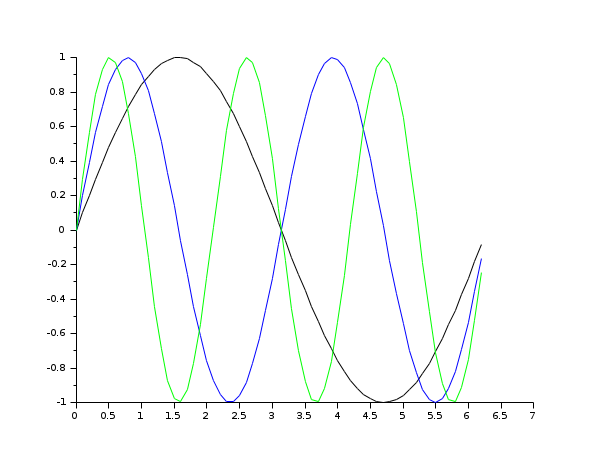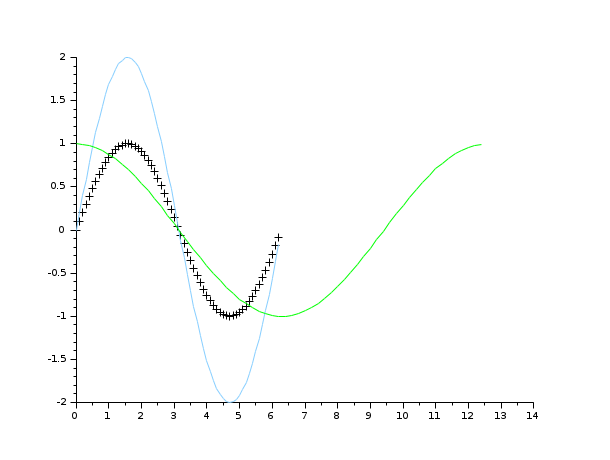Scilab Home page | Wiki | Bug tracker | Forge | Mailing list archives | ATOMS | File exchange
Change language to: Français - Português - 日本語 - Русский

Please note that the recommended version of Scilab is 6.1.1. This page might be outdated.
See the recommended documentation of this function

Scilab help >> Graphics > 2d_plot > plot2d

# plot2d

2D plot

### Calling Sequence

```plot2d([logflag,][x,],y[,style[,strf[,leg[,rect[,nax]]]]])
plot2d([logflag,][x,],y,<opt_args>)```

### Arguments

x

a real matrix or vector. If omitted, it is assumed to be the vector `1:n` where `n` is the number of curve points given by the `y` parameter.

y

a real matrix or vector.

<opt_args>

This represents a sequence of statements `key1=value1,key2=value2`,... where `key1`, `key2,...` can be one of the following:

logflag

sets the scale (linear or logarithmic) along the axes. The associated value should be a string with possible values: `"nn"`, `"nl"` , `"ln"` and `"ll"` .

style

sets the style for each curve. The associated value should be a real vector with integer (positive or negative) values.

strf

controls the display of captions.

`strf` is a string of length 3 `"xyz"` (by default ```strf= "081"``` )

leg

sets the curves captions. The associated value should be a character string.

rect

sets the mimimal bounds requested for the plot. The associated value should be a real vector with four entries: `[xmin,ymin,xmax,ymax]`.

nax

sets the axes labels and tics definition. The associated value should be a real vector with four integer entries `[nx,Nx,ny,Ny].`

frameflag

controls the computation of the actual coordinate ranges from the minimal requested values. The associated value should be an integer ranging from 0 to 8.

axesflag

specifies how the axes are drawn. The associated value should be an integer ranging from 0 to 5.

### Description

`plot2d` plots a set of 2D curves. If you are familiar with Matlab `plot` syntax, you should use plot.

If `x` and `y` are vectors, plot2d(x,y,<opt_args>) plots vector `y` versus vector `x`. `x` and `y` vectors should have the same number of entries.

If `x` is a vector and `y` a matrix plot2d(x,y,<opt_args>) plots each columns of `y` versus vector `x`. In this case the number of columns of `y` should be equal to the number of `x` entries.

If `x` and `y` are matrices, plot2d(x,y,<opt_args>) plots each columns of `y` versus corresponding column of `x`. In this case the `x` and `y` sizes should be the same.

If `y` is a vector, plot2d(y,<opt_args>) plots vector `y` versus vector `1:size(y,'*')`.

If `y` is a matrix, plot2d(y,<opt_args>) plots each columns of `y` versus vector `1:size(y,1)`.

The `<opt_args>` arguments should be used to customize the plot

logflag

This option may be used to set the scale (linear or logarithmic) along the axes. The associated value should be a string with possible values: `"nn"`, `"nl"` , `"ln"` and `"ll"`. `"l"` stands for logarithmic scale and graduations and `"n"` for normal scale.

style

This option may be used to specify how the curves are drawn. If this option is specified, the associated value should be a vector with as many entries as curves.

• if `style(i)` is strictly positive, the curve is drawn as plain line and `style(i)` defines the index of the color used to draw the curve (see getcolor). Note that the line style and the thickness can be set through the polyline entity properties (see polyline_properties).

Piecewise linear interpolation is done between the given curve points.

• if `style(i)` is negative or zero, the given curve points are drawn using marks, `abs(style(i))` defines the mark with id used. Note that the marks color and marks sizes can be set through the polyline entity properties (see polyline_properties).

strf

is a string of length 3 `"xyz"` (by default `strf= "081"`)

x

controls the display of captions.

x=0

no caption.

x=1

captions are displayed. They are given by the optional argument `leg`.

y

controls the computation of the actual coordinate ranges from the minimal requested values. Actual ranges can be larger than minimal requirements.

y=0

no computation, the plot use the previus (or default) scale

y=1

from the rect arg

y=2

from the min/max of the x, y data

y=3

built for an isometric scale from the rect arg

y=4

built for an isometric plot from the min/max of the x, y data

y=5

enlarged for pretty axes from the rect arg

y=6

enlarged for pretty axes from the min/max of the x, y data

y=7

like y=1 but the previus plot(s) are redrawn to use the new scale

y=8

like y=2 but the previus plot(s) are redrawn to use the new scale

z

controls the display of information on the frame around the plot. If axes are requested, the number of tics can be specified by the `nax` optional argument.

z=0

nothing is drawn around the plot.

z=1

axes are drawn, the y=axis is displayed on the left.

z=2

the plot is surrounded by a box without tics.

z=3

axes are drawn, the y=axis is displayed on the right.

z=4

axes are drawn centred in the middle of the frame box.

z=5

axes are drawn so as to cross at point `(0,0)`. If point `(0,0)` does not lie inside the frame, axes will not appear on the graph.

leg

This option may be used to sets the curve captions. It must be a string with the form `"leg1@leg2@...."` where `leg1` , `leg2` , etc. are respectively the captions of the first curve, of the second curve, etc. The default is `" "`.

The curve captions are drawn on below the x-axis. This option is not flexible enough, use the captions or legend functions preferably.

rect

This option may be used to set the mimimal bounds requested for the plot. If this option is specified, the associated value should be a real vector with four entries:

`[xmin,ymin,xmax,ymax]`. `xmin` and `xmax` defines the bounds on the abscissae while `ymin` and `ymax` defines the bounds on the ordinates.

This argument may be used together with the `frameflag` option to specify how the axes boundaries are derived from the given `rect` argument. If the `frameflag` option is not given, it is supposed to be `frameflag=7`.

The axes boundaries can also be customized through the axes entity properties (see axes_properties).

nax

This option may be used to specify the axes labels and tics definition. The associated value should be a real vector with four integer entries `[nx,Nx,ny,Ny]`.

`Nx` gives the number of main tics to be used on the x-axis (to use autoticks set it to -1), `nx` gives the number of subtics to be drawn between two main x-axis tics.

`Ny` and `ny` give similar information for the y-axis.

If `axesflag` option is not set `nax` option supposes that `axesflag` option has been set to 9.

frameflag

This option may be used to control the computation of the actual coordinate ranges from the minimal requested values. Actual ranges can be larger than minimal requirements.

frameflag=0

no computation, the plot use the previous (or default) scale.

frameflag=1

The actual range is the range given by the `rect` option.

frameflag=2

The actual range is computed from the min/max of the `x` and `y` data.

frameflag=3

The actual range is the range given by the `rect` option and enlarged to get an isometric scale.

frameflag=4

The actual range is computed from the min/max of the `x` and `y` data and enlarged to get an isometric scale.

frameflag=5

The actual range is the range given by the `rect` option and enlarged to get pretty axes labels.

frameflag=6

The actual range is computed from the min/max of the `x` and `y` data and enlarged to get pretty axes labels.

frameflag=7

like `frameflag=1` but the previous plot(s) are redrawn to use the new scale. Used to add the current graph to a previous one.

frameflag=8

like`frameflag=2` but the previous plot(s) are redrawn to use the new scale. Used to add the current graph to a previous one.

frameflag=9

like`frameflag=8` but the range is enlarged to get pretty axes labels. This is the default value.

The axes boundaries can also be customized through the axes entity properties (see axes_properties)

axesflag

This option may be used to specify how the axes are drawn. The associated value should be an integer ranging from 0 to 5 :

axesflag=0

nothing is drawn around the plot (axes_visible=["off" "off"];box="off").

axesflag=1

axes are drawn, the y-axis is displayed on the left (axes_visible=["on" "on"];box="on",y_location="left").

axesflag=2

the plot is surrounded by a box without tics (axes_visible=["off" "off"];box="on").

axesflag=3

axes are drawn, the y-axis is displayed on the right (axes_visible=["on" "on"];box="off",y_location="right").

axesflag=4

axes are drawn centered in the middle of the frame, the box being not drawn (axes_visible=["on" "on"];box="off",x_location="middle", y_location="middle").

axesflag=5

axes are drawn centered in the middle of the frame similarly to `axesflag=4`, the difference being that the box is drawn (axes_visible=["on" "on"];box="on",x_location="middle",y_location="middle").

axesflag=9

axes are drawn, the y-axis is displayed on the left (axes_visible=["on" "on"];box="off",y_location="left"). This is the default value

The axes aspect can also be customized through the axes entity properties (see axes_properties).

By default, successive plots are superposed. To clear the previous plot, use `clf()` .

Enter the command `plot2d()` to see a demo.

Other high level plot2d functions exist:

• plot2d2 same as `plot2d` but the curve is supposed to be piecewise constant.

• plot2d3 same as `plot2d` but the curve is plotted with vertical bars.

• plot2d4 same as `plot2d` but the curve is plotted with vertical arrows.

### Examples

```// x initialisation
x=[0:0.1:2*%pi]';
//simple plot
plot2d(sin(x));``````clf();
x=[0:0.1:2*%pi]';
plot2d(x,sin(x));
//multiple plot``````clf();
x=[0:0.1:2*%pi]';
plot2d(x,[sin(x) sin(2*x) sin(3*x)])``````// multiple plot giving the dimensions of the frame
clf();
x=[0:0.1:2*%pi]';
plot2d(x,[sin(x) sin(2*x) sin(3*x)],rect=[0,0,6,0.5]);``````//multiple plot with captions and given tics + style
clf();
x=[0:0.1:2*%pi]';
plot2d(x,[sin(x) sin(2*x) sin(3*x)],..
[1,2,3],leg="L1@L2@L3",nax=[2,10,2,10],rect=[0,-2,2*%pi,2]);``````// isoview
clf();
x=[0:0.1:2*%pi]';
plot2d(x,sin(x),1,frameflag= 4);``````// scale
clf();
x=[0:0.1:2*%pi]';
plot2d(x,sin(x),1,frameflag= 6);``````// auto scaling with previous plots + style
clf();
x=[0:0.1:2*%pi]';
plot2d(x,sin(x),-1);
plot2d(x,2*sin(x),12);
plot2d(2*x,cos(x),3);``````// axis on the right
clf();
x=[0:0.1:2*%pi]';
plot2d(x,sin(x),leg="sin(x)");
a=gca(); // Handle on axes entity
a.y_location ="right";``````// axis centered at (0,0)
clf();
x=[0:0.1:2*%pi]';
plot2d(x-4,sin(x),1,leg="sin(x)");
a=gca(); // Handle on axes entity
a.x_location = "origin";
a.y_location = "origin";
// Some operations on entities created by plot2d ...
a=gca();
a.isoview='on';
a.children // list the children of the axes.
// There are a compound made of two polylines and a legend
poly1= a.children(1).children(1); //store polyline handle into poly1
poly1.foreground = 4; // another way to change the style...
poly1.thickness = 3;  // ...and the tickness of a curve.
poly1.clip_state='off'; // clipping control
leg = a.children(2); // store legend handle into leg
leg.font_style = 9;
leg.line_mode = "on";
a.isoview='off';```• plot — 2D plot
• plot2d1 — 2D plot (logarithmic axes). This function is obsolete.
• plot2d2 — 2D plot (step function)
• plot2d3 — 2D plot (vertical bars)
• plot2d4 — 2D plot (arrows style)
• clf — clear or reset the current graphic figure (window) to default values
• xdel — delete a graphics window
• delete — delete a graphic entity and its children.